# Year 9 Revision - Level 2

This is a level 2 revision exercise to help prepare for Year 9/end of Key Stage 3 tests.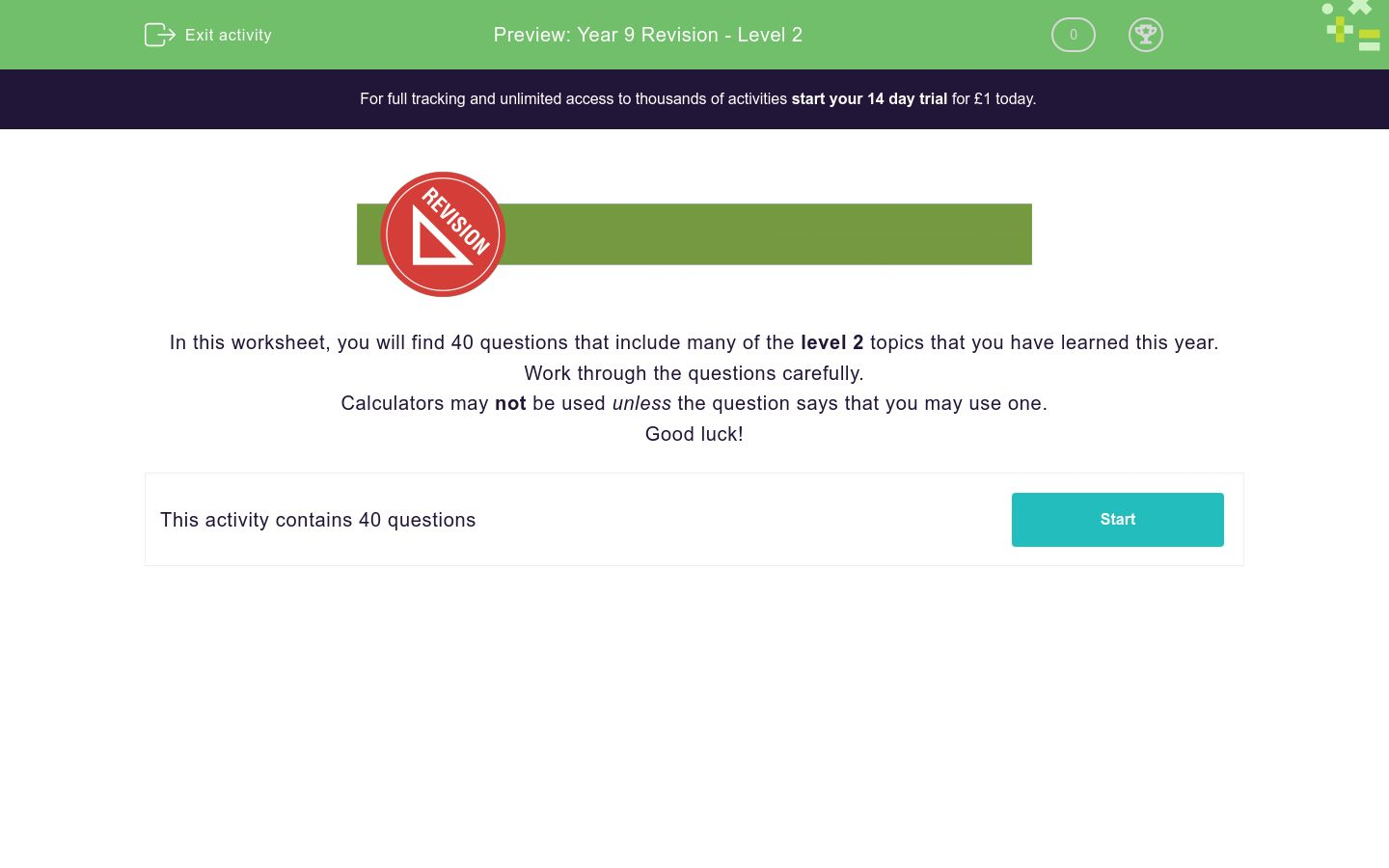Key stage:  KS 3

Curriculum topic:   Revision

Curriculum subtopic:   Year 9 Revision

Difficulty level:### QUESTION 1 of 10In this worksheet, you will find 40 questions that include many of the level 2 topics that you have learned this year.

Work through the questions carefully.

Calculators may not be used unless the question says that you may use one.

Good luck!

Work out:

8403 - 8.403

Work out:

74.32 × 8.4

Which of these sequences will be the first to pass 1000?

A:    3     31     59     87 ...

B:    3     6     9     12 ...

C:    3     9     27     81 ...

A

B

C

By which of the given numbers is the following number divisible?

314 550

2

3

4

5

6

8

9

10

11

Select all the irrational numbers in this list.

0

π

√7

Round the following number to 1, 2 and 3 significant figures.

37255

(Don't leave any space between the numbers.)

 answers 1 sig. fig. 2 sig. figs. 3 sig. figs.

Round the following number to 1, 2 and 3 decimal places.

37.2595

 answers 1 dp 2 dp 3 dp

Write the following number in standard form:

0.000201

2.01 × 10-3

2.01 × 104

2.01 × 10-4

Work out:

 15 - 5 40 24

(Give your answer as a fraction, reduced to its lowest terms, e.g. 7/10.)

Work out:

 15 ÷ 18 40 24

(Give your answer as a fraction, reduced to its lowest terms, e.g. 7/10.)

Match the fractions to their decimal equivalents.

## Column B

6/8
0.125
1/8
0.5
4/8
0.75
9/12
0.375
3/8
0.75

Work out:

29.694 ÷ 0.0021

Work out:

 ( 1 )7 2

Round each of these numbers to 1 sig. fig. to work out an approximate answer to:

 29.1 × 41.9 57.8

Find the original price of a television in pounds, sold for £320 after a 20% reduction in a sale.

(Just write the number.)

Jane sells 120 cakes on Monday.

She sells 102 cakes on Tuesday.

What is the percentage decrease in sales from Monday to Tuesday?

(Just write the number without the % sign.)

Work out the nth term in this sequence and write the formula in the answer box:

-2, 5, 12, 19, 26 ...

Find the first 5 terms in the sequence given by the formula:

Tn = 5n2

where n is the position of the term in the sequence.

 value T1 T2 T3 T4 T5

Form an equation from the angles shown in this triangle and solve it to find the value of a.

(Just write the number.)Simplify:

(-3a + 1) - (a - 3)

Simplify:

2a3b × 3a4b3

5a7b

6a7b3

6a7b4

Multiply out the two brackets and simplify:

(2x - 6)(4x + 6)

8x2 - 12x - 36

8x2 + 36x - 12

Simplify:

(p3q4)7

p10q11

p21q11

p21q28

Solve for a:

a -16 - 2a = a - 21 + 3a

(Just write the value of a as a number.)

Solve simultaneously:

6x + y = 16

3x + y = 10

Solve the following equation:

 a - a = 14 3 12

(Just write the value of a.)

State the gradient of the line with equation:

y = 4 - 7x

Use the graph to select the best estimate for the solution to the simultaneous equations:

y = 2x - 2

y = -1 - x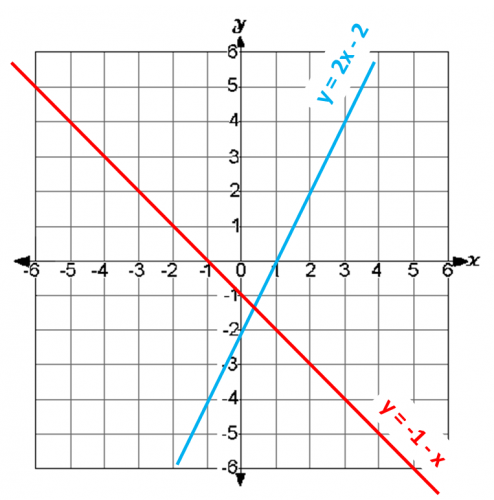x = 0.3, y = 1.3

x = 3.3, y = -1.3

x = 0.3, y = -1.3

A sum of money is shared in the ratio 7:5 between two people, A and B.

If A gets £84, how much money is there in total in £?

(Just write the number.)

A plane flies from London to Rome at an average speed of 668 km/h. The flying time is 2¼ hours.

Find the distance travelled by the plane in km.

(Just write the number.)You may use a calculator for this question.

Find the area in cm2 of a circle of diameter 6.9 cm.

(Just write the number to 1 decimal place.)

A regular polygon has 18 sides.

Calculate its interior angle.

(Just write the number.)You may use a calculator for this question.

Find the surface area of this cylinder in cm2 to 3 sig. figs.

(Just write the number.)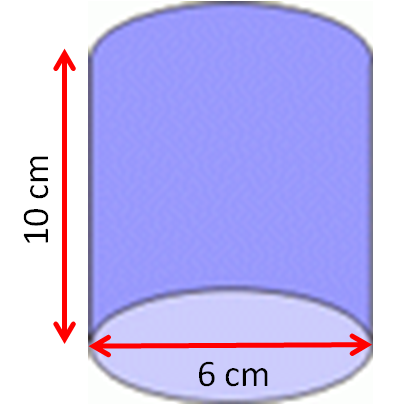You may use a calculator for this question.

Use Pythagoras' Theorem to calculate the length of the side, a, in the following right-angled triangle.You may use a calculator for this question.

Using trigonometry, calculate the side length x to 3 sig. figs.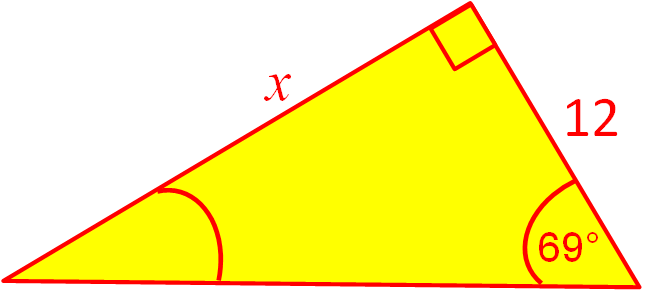You may use a calculator for this question.

Using trigonometry, calculate the angle θº to 3 sig. figs.

(Just write the number.)Two fair dice are thrown.Make a list of the possible outcomes on a piece of paper.

1   1

1   2

1   3

1   4

1   5

1   6

2   1

2   2

2   3

2   4... etc.

What is the probability of getting two even numbers?

(Write your answer with the / symbol, e.g. 2/3 and remember to reduce your fraction to its lowest terms.)

The tree diagram shows the probabilities that my bus is on time or late on Friday and Saturday.

Buses are usually late on Saturdays because it is market day.

Calculate the probability that it will be on time on one day and late on the other.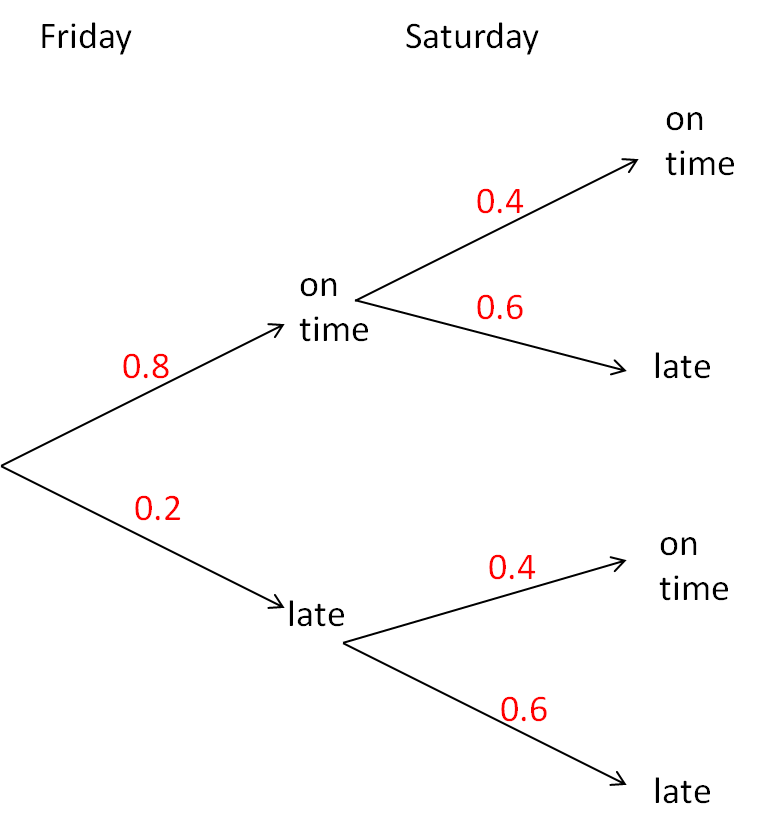You may use a calculator for this question.

This table shows the number of pets owned by children in a Year 9 class at school.

Find the mean number of pets owned by a child.

Number of pets 0  1   2   3
Frequency 11 8 3 8

This chart shows the heights of children in a Year 9 class at school.

Find the median class.110 cm to 115 cm

115 cm to 120 cm

120 cm to 125 cm

125 cm to 130 cm

130 cm to 135 cm

135 cm to 140 cm

140 cm to 145 cm

145 cm to 150 cm

150 cm to 155 cm

• Question 1

Work out:

8403 - 8.403

8394.597
EDDIE SAYS
Change 8403 to 8403.000.
Line up the decimal points and subtract the digits in columns, borrowing if necessary.
• Question 2

Work out:

74.32 × 8.4

624.288
EDDIE SAYS
Use long multiplication to work out 7432 × 84.
Then divide the answer by 1000.
• Question 3

Which of these sequences will be the first to pass 1000?

A:    3     31     59     87 ...

B:    3     6     9     12 ...

C:    3     9     27     81 ...

C
EDDIE SAYS
C shows the powers of 3, which increase very quickly.
The next number is 243, then 729.
The next number will be more than 1000.
• Question 4

By which of the given numbers is the following number divisible?

314 550

2
3
5
6
9
10
EDDIE SAYS
2: ends in even digit.
3: digit sum is 18, which is divisible by 3.
4: last two-digit number is not divisible by 4.
5: ends in 0 or 5.
6: divisible by 2 and by 3.
8: last three-digit number is not divisible by 8.
9: digit sum is 18, which is divisible by 9.
10: ends in 0.
11: compare alternate digit sums which are different.
• Question 5

Select all the irrational numbers in this list.

π
√7
EDDIE SAYS
0 and ⅓ are rational because they can be written as fractions.
π and √7 are never-ending, non-recurring decimals.
• Question 6

Round the following number to 1, 2 and 3 significant figures.

37255

(Don't leave any space between the numbers.)

 answers 1 sig. fig. 2 sig. figs. 3 sig. figs.
EDDIE SAYS
1 sig. fig: 37255 is between 30000 and 40000 but nearer to 40000.
2 sig. figs: 37255 is between 37000 and 38000 but nearer to 37000.
3 sig. figs: 37255 is between 37200 and 37300 but nearer to 37300.
• Question 7

Round the following number to 1, 2 and 3 decimal places.

37.2595

 answers 1 dp 2 dp 3 dp
EDDIE SAYS
1 dp: 37.2595 is between 37.2 and 37.3 but nearer to 37.3.
2 dp: 37.2595 is between 37.25 and 37.26 but nearer to 37.26.
3 dp: 37.2595 is between 37.259 and 37.260 but nearer to 37.260.
• Question 8

Write the following number in standard form:

0.000201

2.01 × 10-4
EDDIE SAYS
Fix the decimal point after the first non-zero digit, then count how many times you must divide by 10 to get back to 0.000201. The index will be negative.
• Question 9

Work out:

 15 - 5 40 24

(Give your answer as a fraction, reduced to its lowest terms, e.g. 7/10.)

1/6
EDDIE SAYS
45/120 - 25/120 = 20/120 = 1/6
• Question 10

Work out:

 15 ÷ 18 40 24

(Give your answer as a fraction, reduced to its lowest terms, e.g. 7/10.)

1/2
EDDIE SAYS
Turn second fraction upside down and multiply.
15/40 × 24/18
Reduce to get 3/8 × 4/3 = 4/8 = 1/2.
• Question 11

Match the fractions to their decimal equivalents.

## Column B

6/8
0.75
1/8
0.125
4/8
0.5
9/12
0.75
3/8
0.375
EDDIE SAYS
6/8 = 3/4 = 0.75
1/8 = 1.000 ÷ 8 = 0.125
4/8 = 1/2 = 0.5
9/12 = 3/4 = 0.75
3/8 = 3.000 ÷ 8 = 0.375
• Question 12

Work out:

29.694 ÷ 0.0021

14140
14 140
EDDIE SAYS
Multiply both numbers by 10 000 to get:
296 940 ÷ 21
Use long division, or divide by 3 and 7 to get the answer.
• Question 13

Work out:

 ( 1 )7 2

1/128
EDDIE SAYS
27 = 128
• Question 14

Round each of these numbers to 1 sig. fig. to work out an approximate answer to:

 29.1 × 41.9 57.8
20
EDDIE SAYS
30 × 40 = 1200
1200 ÷ 60 = 20
• Question 15

Find the original price of a television in pounds, sold for £320 after a 20% reduction in a sale.

(Just write the number.)

400
EDDIE SAYS
320 ÷ 0.8 = 400
• Question 16

Jane sells 120 cakes on Monday.

She sells 102 cakes on Tuesday.

What is the percentage decrease in sales from Monday to Tuesday?

(Just write the number without the % sign.)

15
EDDIE SAYS
Actual decrease = 120 - 102 = 18
% decrease = 18 ÷ 120 × 100 = 15
• Question 17

Work out the nth term in this sequence and write the formula in the answer box:

-2, 5, 12, 19, 26 ...

7n-9
7n - 9
EDDIE SAYS
Common difference is 7.
• Question 18

Find the first 5 terms in the sequence given by the formula:

Tn = 5n2

where n is the position of the term in the sequence.

 value T1 T2 T3 T4 T5
EDDIE SAYS
T1 = 5 × 12 = 5 × 1 = 5
T2 = 5 × 22 = 5 × 4 = 20
T3 = 5 × 32 = 5 × 9 = 45
T4 = 5 × 42 = 5 × 16 = 80
T5 = 5 × 52 = 5 × 25 = 125
• Question 19

Form an equation from the angles shown in this triangle and solve it to find the value of a.

(Just write the number.)20
EDDIE SAYS
60 - a + 3a + 30 + 4a - 30 = 180
60 + 6a = 180
6a = 120
a = 20
• Question 20

Simplify:

(-3a + 1) - (a - 3)

-4a+4
-4a + 4
4-4a
4 - 4a
EDDIE SAYS
-3a + 1 - a + 3
• Question 21

Simplify:

2a3b × 3a4b3

6a7b4
EDDIE SAYS
2 × 3 × a × a × a × a × a × a × a × b × b × b × b
• Question 22

Multiply out the two brackets and simplify:

(2x - 6)(4x + 6)

8x2 - 12x - 36
EDDIE SAYS
2x(4x + 6) - 6(4x + 6) = 8x2 + 12x - 24x - 36
• Question 23

Simplify:

(p3q4)7

p21q28
EDDIE SAYS
p3q4 × p3q4 × p3q4 × p3q4 × p3q4 × p3q4 × p3q4
p3 × p3 × p3 × p3 × p3 × p3 × p3 × q4 × q4 × q4 × q4 × q4 × q4 × q4
• Question 24

Solve for a:

a -16 - 2a = a - 21 + 3a

(Just write the value of a as a number.)

1
EDDIE SAYS
-16 - a = 4a - 21
-a - 4a = -21 + 16
-5a = -5
a = 1
• Question 25

Solve simultaneously:

6x + y = 16

3x + y = 10

EDDIE SAYS
Subtract the equations to get:
3x = 6
x = 2
y = 10 - 3x = 10 - 6 = 4
• Question 26

Solve the following equation:

 a - a = 14 3 12

(Just write the value of a.)

56
EDDIE SAYS
Multiply both sides by the denominator 12.
4a - a = 168
3a = 168
a = 168 ÷ 3 = 56
• Question 27

State the gradient of the line with equation:

y = 4 - 7x

-7
- 7
EDDIE SAYS
The gradient is m, when in the form y = mx + c.
The coefficient of x is -7 here.
• Question 28

Use the graph to select the best estimate for the solution to the simultaneous equations:

y = 2x - 2

y = -1 - xx = 0.3, y = -1.3
EDDIE SAYS• Question 29

A sum of money is shared in the ratio 7:5 between two people, A and B.

If A gets £84, how much money is there in total in £?

(Just write the number.)

144
EDDIE SAYS
There are £84 in 7 bags, so each bag contains £84 ÷ 7 = £12.
In total, there are 12 bags, i.e. 12 × £12 = £144.
• Question 30

A plane flies from London to Rome at an average speed of 668 km/h. The flying time is 2¼ hours.

Find the distance travelled by the plane in km.

(Just write the number.)

1503
EDDIE SAYS
668 × 2¼ = 668 + 668 + 668 ÷ 4 = 668 + 668 + 167
• Question 31You may use a calculator for this question.

Find the area in cm2 of a circle of diameter 6.9 cm.

(Just write the number to 1 decimal place.)

37.4
EDDIE SAYS
Radius = 6.9 ÷ 2 = 3.45 cm
Area = π × r2 = π × 3.452 = 37.3928...
• Question 32

A regular polygon has 18 sides.

Calculate its interior angle.

(Just write the number.)

160
EDDIE SAYS
180 - 360 ÷ 18 = 180 - 20
• Question 33You may use a calculator for this question.

Find the surface area of this cylinder in cm2 to 3 sig. figs.

(Just write the number.)245
EDDIE SAYS
Two circles and a rectangle.
The base of the rectangle is the circumference of the circle.
2 × π × 3² + 6 × π × 10
• Question 34You may use a calculator for this question.

Use Pythagoras' Theorem to calculate the length of the side, a, in the following right-angled triangle.5.66
EDDIE SAYS
a = √(92 - 72) = √32 = 5.6568...
• Question 35You may use a calculator for this question.

Using trigonometry, calculate the side length x to 3 sig. figs.31.3
EDDIE SAYS
x = 12 × tan69°
• Question 36You may use a calculator for this question.

Using trigonometry, calculate the angle θº to 3 sig. figs.

(Just write the number.)38.9
EDDIE SAYS
θ = cos-1(7/9)
• Question 37

Two fair dice are thrown.Make a list of the possible outcomes on a piece of paper.

1   1

1   2

1   3

1   4

1   5

1   6

2   1

2   2

2   3

2   4... etc.

What is the probability of getting two even numbers?

(Write your answer with the / symbol, e.g. 2/3 and remember to reduce your fraction to its lowest terms.)

1/4
EDDIE SAYS
Out of 36 outcomes, 9 have two even numbers.
2 2, 2 4, 2 6
4 2, 4 4, 4 6
6 2, 6 4, 6 6
• Question 38

The tree diagram shows the probabilities that my bus is on time or late on Friday and Saturday.

Buses are usually late on Saturdays because it is market day.

Calculate the probability that it will be on time on one day and late on the other.0.56
EDDIE SAYS
0.8 × 0.6 + 0.2 × 0.4 = 0.48 + 0.08 = 0.56
• Question 39You may use a calculator for this question.

This table shows the number of pets owned by children in a Year 9 class at school.

Find the mean number of pets owned by a child.

Number of pets 0  1   2   3
Frequency 11 8 3 8

1.3
EDDIE SAYS
Total no. of pets = 0 × 11 + 1 × 8 + 2 × 3 + 3 × 8 = 8 + 6 + 24 = 38
Total no. of children = 11 + 8 + 3 + 8 = 30
Mean = 38 ÷ 30 = 1.266666...
• Question 40

This chart shows the heights of children in a Year 9 class at school.

Find the median class.130 cm to 135 cm
EDDIE SAYS
No. of children in class = 1 + 3 + 7 + 5 + 4 + 3 + 1 = 24
We need to find the 12th and 13th child in order of height.
They will both be in the 130 cm to 135 cm group, because the first 11 children are in the first 3 groups shown.
---- OR ----

Sign up for a £1 trial so you can track and measure your child's progress on this activity.

### What is EdPlace?

We're your National Curriculum aligned online education content provider helping each child succeed in English, maths and science from year 1 to GCSE. With an EdPlace account you’ll be able to track and measure progress, helping each child achieve their best. We build confidence and attainment by personalising each child’s learning at a level that suits them.

Get started